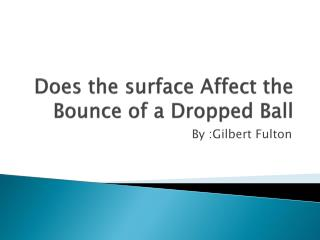# Does the surface Affect the Bounce of a Dropped Ball - PowerPoint PPT PresentationDownload PresentationDoes the surface Affect the Bounce of a Dropped Ball

Does the surface Affect the Bounce of a Dropped BallDownload Presentation## Does the surface Affect the Bounce of a Dropped Ball

- - - - - - - - - - - - - - - - - - - - - - - - - - - E N D - - - - - - - - - - - - - - - - - - - - - - - - - - -
##### Presentation Transcript

1. Does the surface Affectthe Bounce of a Dropped Ball By :Gilbert Fulton

2. Hypothesis • I hypothesize that the sand will have the most affect on the dropped ball

3. Variables • Independent Variable: The type of surface the ball is being dropped on • Dependent Variable: The height of the ball after being dropped on the surface

4. Controlled Variables • The height the ball is dropped • The same ball • The same conditions when I experiment • The same amount of force • The same metric units used

5. Research Paper • Potential energy • Elastic • Friction • Air resistance • Gravity

6. Conclusion • The sand did have the most affect on the dropped ball ,so my hypothesis was correct.

7. Does the Type of Ball Affect the Bounce of a dropped Ball By: Gilbert Fulton I hypothesize that the soccer ball will have the most affect on the dropped ball.

8. Hypothesis • I hypothesize that the soccer ball will have the most affect.

9. Variables • Independent variable: The type of ball. • Dependent variable: Does the type affect the bounce of a dropped ball.

10. Controlled Variables • The height the ball is being dropped at • The type of surface • The same conditions while testing • The same force being used • The metric units used

11. Research Paper • Potential energy • Elastic • Air resistance • Gravity • Friction

12. Conclusion • The soccer ball had the most affect and my hypothesis was correct.

13. Does the Different Size Affect the Bounce of a Dropped Ball? By: Gilbert Fulton

14. Hypothesis • I hypothesize that the football will have the most affect

15. Variables • Independent variable: The sizes of the ball • Dependent variable: The affect of the ball being dropped

16. Controlled Variables • The type of surface • The height the ball is being dropped at • The same metric units used • The same force applied • Experimenting in the same conditions

17. Research Paper • Elastic • Gravity • Air resistance • Friction • Potential energy

18. Conclusion • The basketball had the most affect and my hypothesis was incorrect.